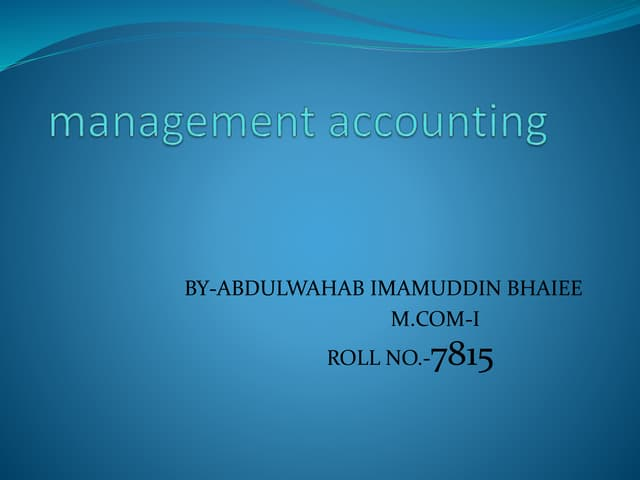Management accounting
1. (Kelated to checkpoint 4.1) (Liquidity analyals) The most recent bolance sheet of Raconteurs, inc, (a milions) is found here: a. Calculate Raconteurs' current ratio and acid-test (quick) ratio. b. Benchmark ratios for the current and acid-test (quick) ratio are 1.50 and 1.20 , respectively. What can you say about the liquidty of Racontour's operations based on these two ratios? a. Calculate Raconteurs' current ratio and acld-lest (quick) ratio. Raconteurs' curreot ratio is (Round to two decimal piaces.) Raconteurs' acid-test ratio is (Round to two decimal places.) b. Benchmark tatios for the current and acid-test (quick) ratio ace 1,50 and 1,20 , retpectively. What can you say about the liquidty of Raconiours operations based on these two rates 7 (seliset at the correct choices from below.) A. Based on its current rabo, Raconteurs, Inc, is sightly less liquid thas the peer group since its current rato is higher. B. Raconteurst acid-test mitio indicates highee liquidty than its current rato and Raconteurs adidetest ratio show higher liquidity when compared aganat the industy average. C. Raconteurs' acid-test rato indicates lower liquidity than is current rato and Raconteur's aod-test rato shows lower liquidty when compared againat the indistry arerape. D. Aased on its current ratio, Raconteurs, inc. Is alightly more liguid than the peer group shen its current rato ia higher. Current assets begin{tabular}{lr} Cash and marketable securities & $10.0 Accounts receivable &$40.0 Inventory & $60.0 cline { 2 - 2 } Total current assets &$110.0 end{tabular} Current liabilities begin{tabular}{lr} Accrued wages and taxes & $5.0 Accounts payable &$35.0 Notes payable & $30.0 cline { 2 - 2 } Total current liabilities &$70.0 hline end{tabular} (Click on the icon in order to copy its contents into a spreadsheet.) Done